Social Question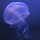Can you figure out this basic symbolic reasoning puzzle?

Asked by SpookyHeather (24) July 18th, 2021
6 responses
“Great Question” (1)

if ::?a:: then e
if :?a: then e
if ::o?:: then i
if :o?: then i
if ::?o:: then ?

Bonus
if :?i: then ?Follow this questionSend to a friend!Topics: ,
Observing members: 0Composing members: 0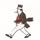Nope

stanleybmanly (24128)“Great Answer” (2)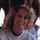Uh. No.

Dutchess_III (44682)“Great Answer” (0)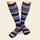Maybe if I knew what the symbols meant, I could. It’s apparently not about logic or reasoning, though, it’s about notation. So it’s sort of like asking us if we can answer this riddle:

Γιατί η κότα διέσχισε τον δρόμο?

kneesox (4330)“Great Answer” (0)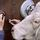u

bonus: o

did I win?

Smashley (9000)“Great Answer” (3)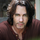I agree with @Smashley.

The first four examples tell us that there is no relevant difference between ::[x]:: and :[x]: because they both return the same result (and if there is a relevant difference, then we have been given insufficient information to solve the puzzle).

In the first two examples, the question mark is before the letter, and the result is the next letter in the vowel series {a, e, i, o, u}. In the last two examples, the question mark is after the letter, and the result is the previous letter in the vowel series {a, e, i, o, u}.

This pattern is established by the examples and not contradicted by the examples, and so it is at least a solution. And since a good puzzle only has one solution, the answers are either “u” and “o,” or there is not a unique solution to the puzzle (making it a bad puzzle).

One might be worried about a potential trick stemming from the reuse of a question mark. But the existence of the bonus rules this out (assuming, again, that it is not a bad puzzle).

JeSuisRickSpringfield (7341)“Great Answer” (1)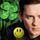u
bonus o
I interpret it as ?v to mean the vowel following the vowel. v? means the vowel before the vowel.

I agree with @Smashley @JeSuisRickSpringfield

LuckyGuy (40294)“Great Answer” (1)